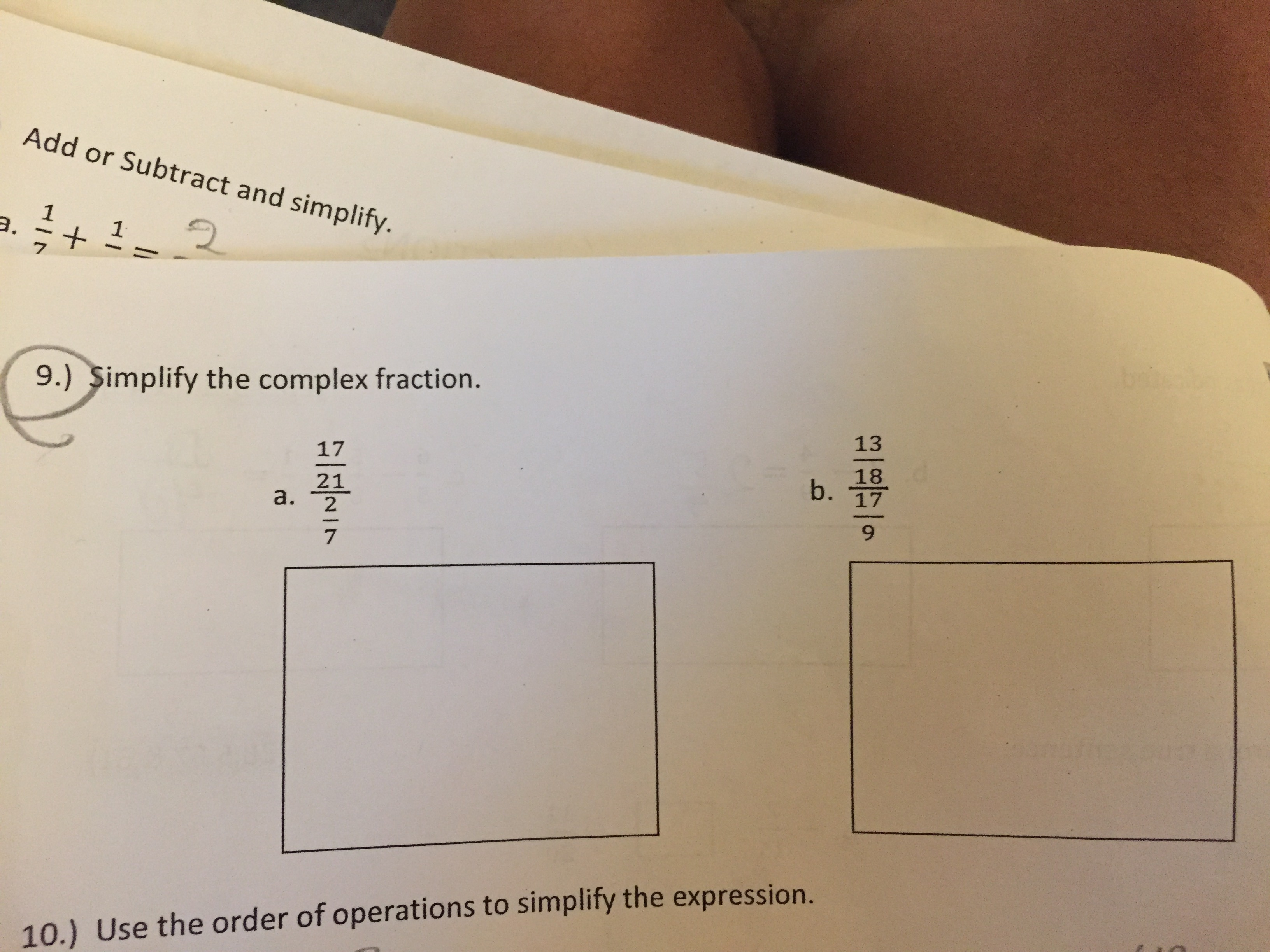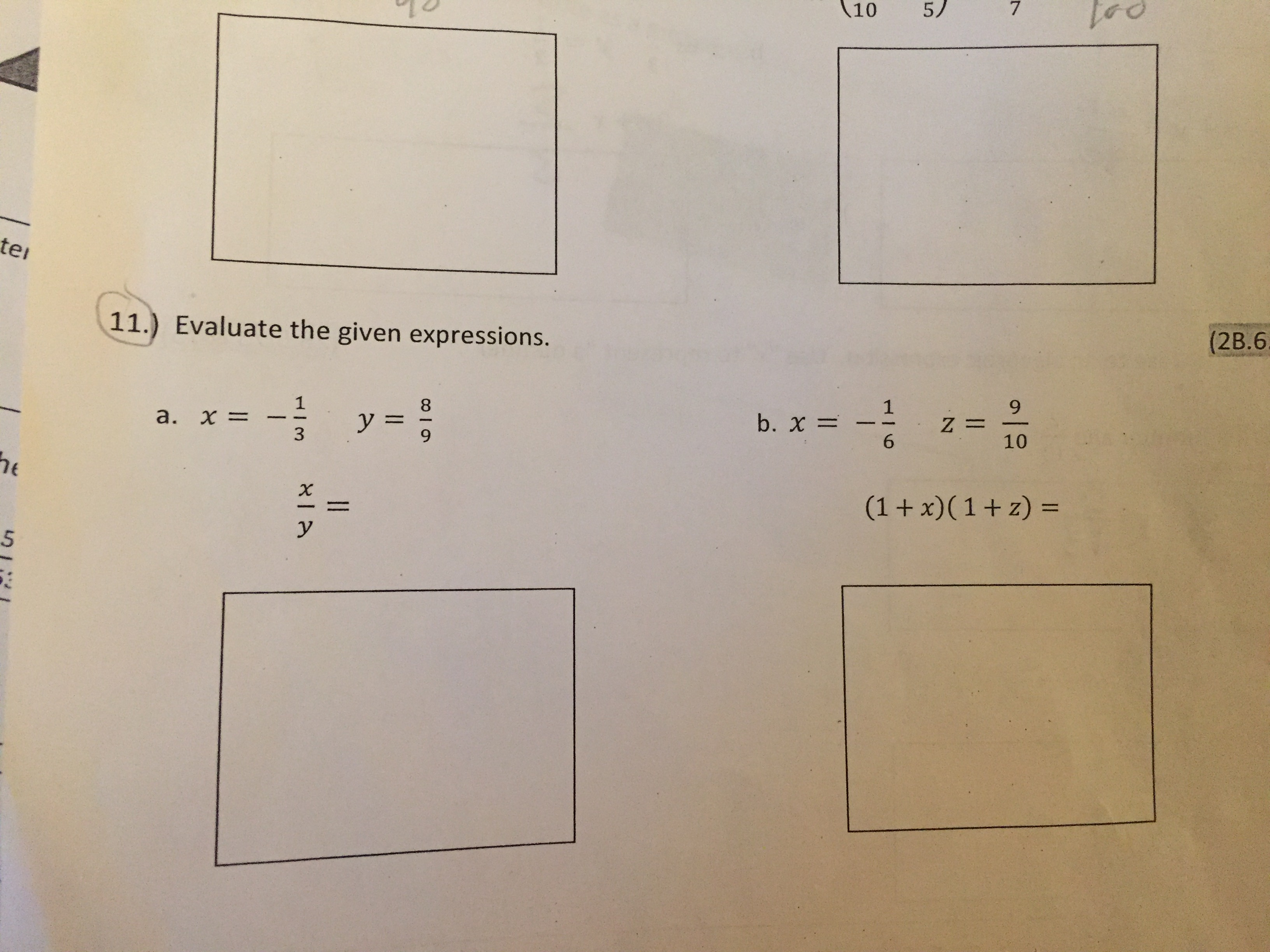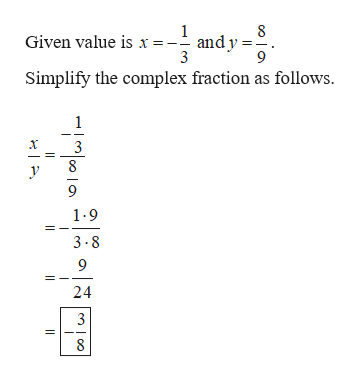# Add or Subtract and simplifya.19.) Simplify the complex fraction.131718b.1721a.2710.) Use the order of operations to simplify the expression. 75/10tei(2B.611.) Evaluate the given expressions.18= Z10b. x=y =a. x=63he(1+ x)(1+z)XyIlHIm

Question
1 views

I need help with thishelp_outlineImage TranscriptioncloseAdd or Subtract and simplify a. 1 9.) Simplify the complex fraction. 13 17 18 b. 17 21 a. 2 7 10.) Use the order of operations to simplify the expression. fullscreenhelp_outlineImage Transcriptionclose7 5/ 10 tei (2B.6 11.) Evaluate the given expressions. 1 8 = Z 10 b. x= y = a. x= 6 3 he (1+ x)(1+z) X y Il HIm fullscreen
check_circle

Step 1

9.)-a.

Simplify the complex fraction as follows.

Step 2

9.)-b.

Simplify the complex fraction as follows.

Step 3

11.a.

Evaluate the given...help_outlineImage Transcriptionclose8 and y 9 Given value is x= Simplify the complex fraction as follows 1 3 8 9 1-9 3-8 9 24 8 fullscreen

### Want to see the full answer?

See Solution

#### Want to see this answer and more?

Solutions are written by subject experts who are available 24/7. Questions are typically answered within 1 hour.*

See Solution
*Response times may vary by subject and question.
Tagged in

### Algebra